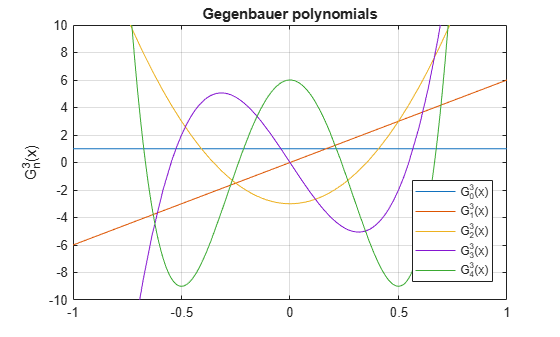# gegenbauerC

Gegenbauer polynomials

## Syntax

``gegenbauerC(n,a,x)``

## Description

example

````gegenbauerC(n,a,x)` represents the `n`th-degree Gegenbauer (ultraspherical) polynomial with parameter `a` at the point `x`.```

## Examples

### First Four Gegenbauer Polynomials

Find the first four Gegenbauer polynomials for the parameter `a` and variable `x`.

```syms a x gegenbauerC([0, 1, 2, 3], a, x)```
```ans = [ 1, 2*a*x, (2*a^2 + 2*a)*x^2 - a,... ((4*a^3)/3 + 4*a^2 + (8*a)/3)*x^3 + (- 2*a^2 - 2*a)*x]```

### Gegenbauer Polynomials for Numeric and Symbolic Arguments

Depending on its arguments, `gegenbauerC` returns floating-point or exact symbolic results.

Find the value of the fifth-degree Gegenbauer polynomial for the parameter ```a = 1/3``` at these points. Because these numbers are not symbolic objects, `gegenbauerC` returns floating-point results.

`gegenbauerC(5, 1/3, [1/6, 1/4, 1/3, 1/2, 2/3, 3/4])`
```ans = 0.1520 0.1911 0.1914 0.0672 -0.1483 -0.2188```

Find the value of the fifth-degree Gegenbauer polynomial for the same numbers converted to symbolic objects. For symbolic numbers, `gegenbauerC` returns exact symbolic results.

`gegenbauerC(5, 1/3, sym([1/6, 1/4, 1/3, 1/2, 2/3, 3/4]))`
```ans = [ 26929/177147, 4459/23328, 33908/177147, 49/729, -26264/177147, -7/32]```

### Evaluate Chebyshev Polynomials with Floating-Point Numbers

Floating-point evaluation of Gegenbauer polynomials by direct calls of `gegenbauerC` is numerically stable. However, first computing the polynomial using a symbolic variable, and then substituting variable-precision values into this expression can be numerically unstable.

Find the value of the 500th-degree Gegenbauer polynomial for the parameter `4` at `1/3` and `vpa(1/3)`. Floating-point evaluation is numerically stable.

```gegenbauerC(500, 4, 1/3) gegenbauerC(500, 4, vpa(1/3))```
```ans = -1.9161e+05 ans = -191609.10250897532784888518393655```

Now, find the symbolic polynomial `C500 = gegenbauerC(500, 4, x)`, and substitute `x = vpa(1/3)` into the result. This approach is numerically unstable.

```syms x C500 = gegenbauerC(500, 4, x); subs(C500, x, vpa(1/3))```
```ans = -8.0178726380235741521208852037291e+35```

Approximate the polynomial coefficients by using `vpa`, and then substitute `x = sym(1/3)` into the result. This approach is also numerically unstable.

`subs(vpa(C500), x, sym(1/3))`
```ans = -8.1125412405858470246887213923167e+36```

### Plot Gegenbauer Polynomials

Plot the first five Gegenbauer polynomials for the parameter `a = 3`.

```syms x y fplot(gegenbauerC(0:4,3,x)) axis([-1 1 -10 10]) grid on ylabel('G_n^3(x)') title('Gegenbauer polynomials') legend('G_0^3(x)', 'G_1^3(x)', 'G_2^3(x)', 'G_3^3(x)', 'G_4^3(x)',... 'Location', 'Best')```## Input Arguments

collapse all

Degree of the polynomial, specified as a nonnegative integer, symbolic variable, expression, or function, or as a vector or matrix of numbers, symbolic numbers, variables, expressions, or functions.

Parameter, specified as a nonnegative integer, symbolic variable, expression, or function, or as a vector or matrix of numbers, symbolic numbers, variables, expressions, or functions.

Evaluation point, specified as a number, symbolic number, variable, expression, or function, or as a vector or matrix of numbers, symbolic numbers, variables, expressions, or functions.

collapse all

### Gegenbauer Polynomials

• Gegenbauer polynomials are defined by this recursion formula.

`$G\left(0,a,x\right)=1,\text{ }G\left(1,a,x\right)=2ax,\text{ }G\left(n,a,x\right)=\frac{2x\left(n+a-1\right)}{n}G\left(n-1,a,x\right)-\frac{n+2a-2}{n}G\left(n-2,a,x\right)$`

• For all real a > -1/2, Gegenbauer polynomials are orthogonal on the interval -1 ≤ x ≤ 1 with respect to the weight function $w\left(x\right)={\left(1-{x}^{2}\right)}^{a-\frac{1}{2}}$.

• Chebyshev polynomials of the first and second kinds are special cases of the Gegenbauer polynomials.

`$U\left(n,x\right)=G\left(n,1,x\right)$`
• Legendre polynomials are also a special case of the Gegenbauer polynomials.

`$P\left(n,x\right)=G\left(n,\frac{1}{2},x\right)$`

## Tips

• `gegenbauerC` returns floating-point results for numeric arguments that are not symbolic objects.

• `gegenbauerC` acts element-wise on nonscalar inputs.

• All nonscalar arguments must have the same size. If one or two input arguments are nonscalar, then `gegenbauerC` expands the scalars into vectors or matrices of the same size as the nonscalar arguments, with all elements equal to the corresponding scalar.

 Hochstrasser, U. W. “Orthogonal Polynomials.” Handbook of Mathematical Functions with Formulas, Graphs, and Mathematical Tables. (M. Abramowitz and I. A. Stegun, eds.). New York: Dover, 1972.

 Cohl, Howard S., and Connor MacKenzie. “Generalizations and Specializations of Generating Functions for Jacobi, Gegenbauer, Chebyshev and Legendre Polynomials with Definite Integrals.” Journal of Classical Analysis, no. 1 (2013): 17–33. https://doi.org/10.7153/jca-03-02.